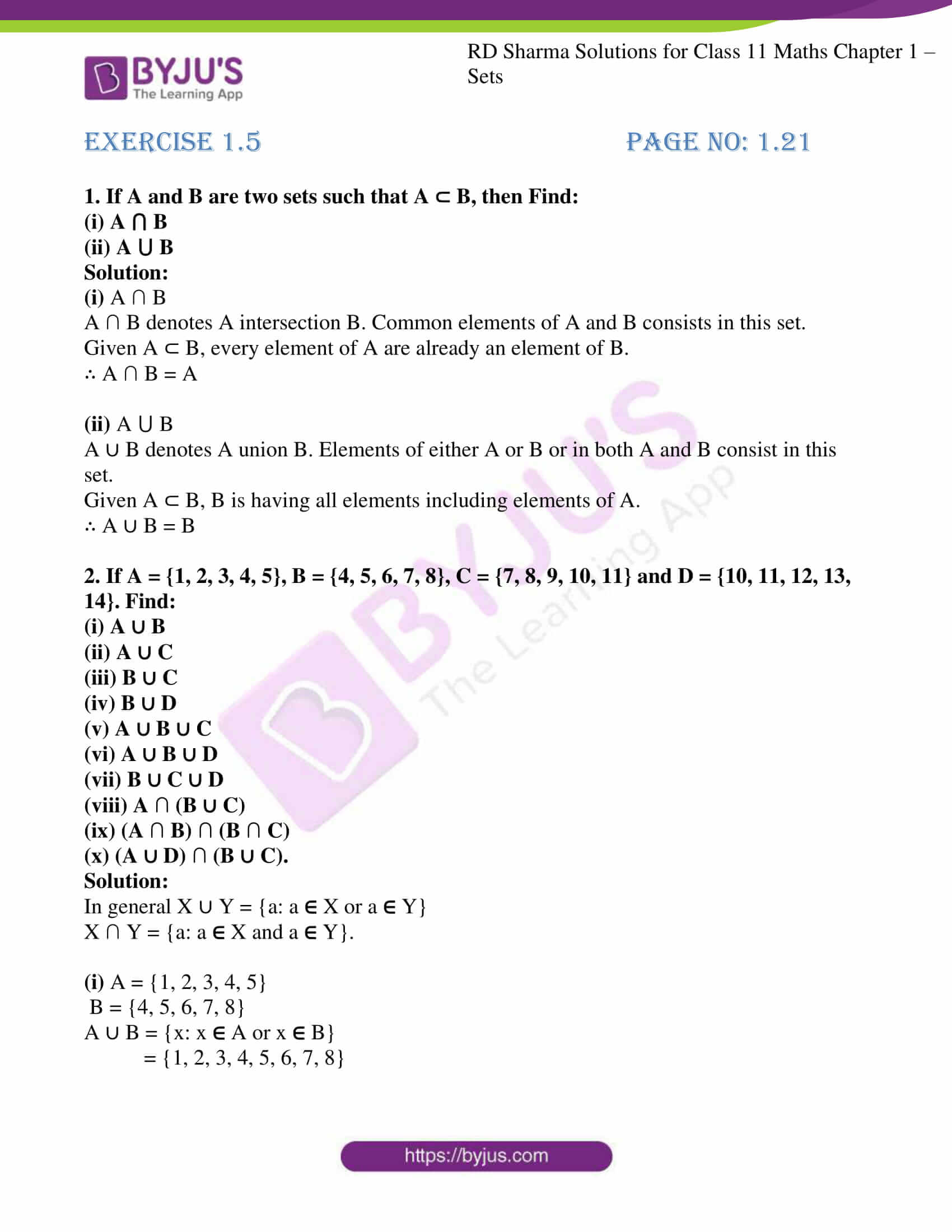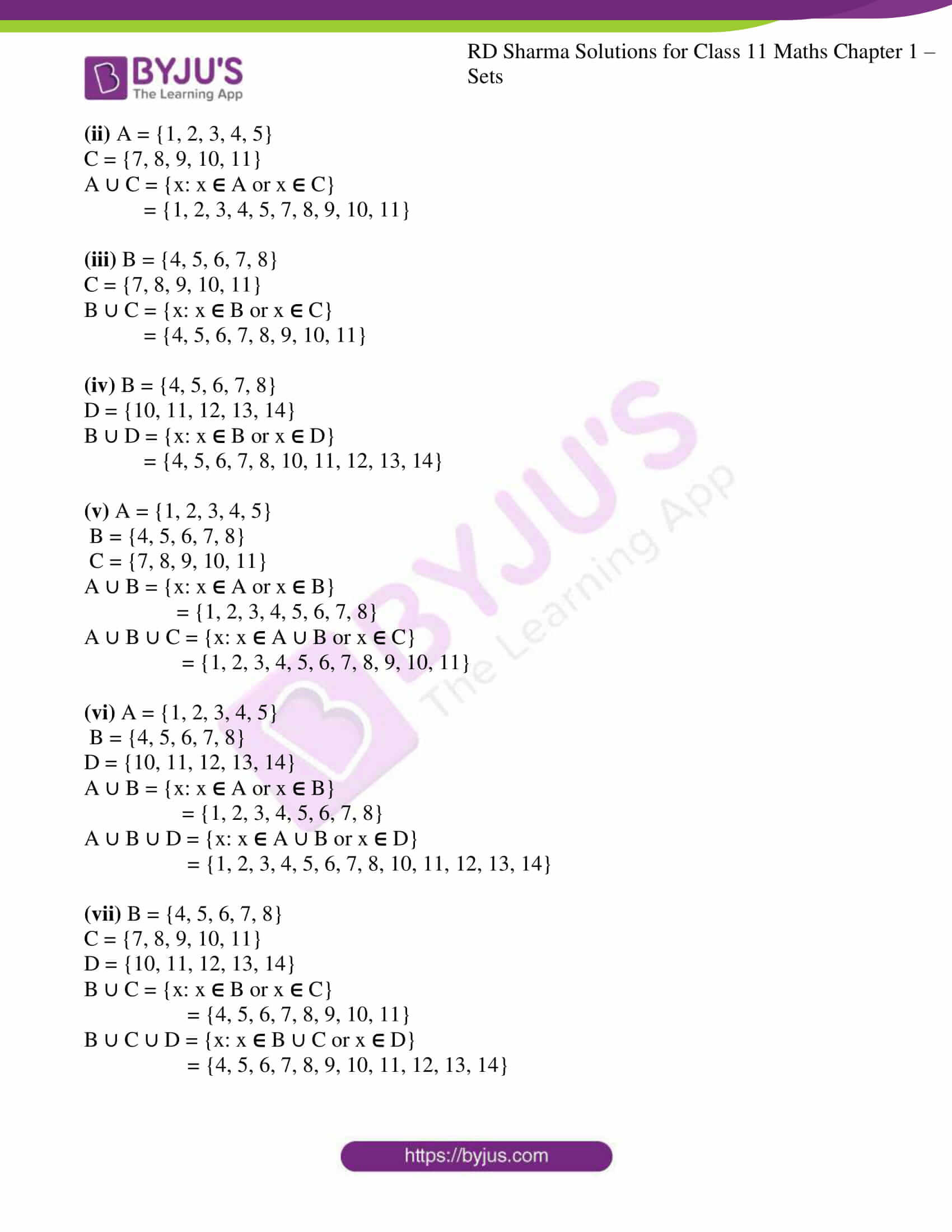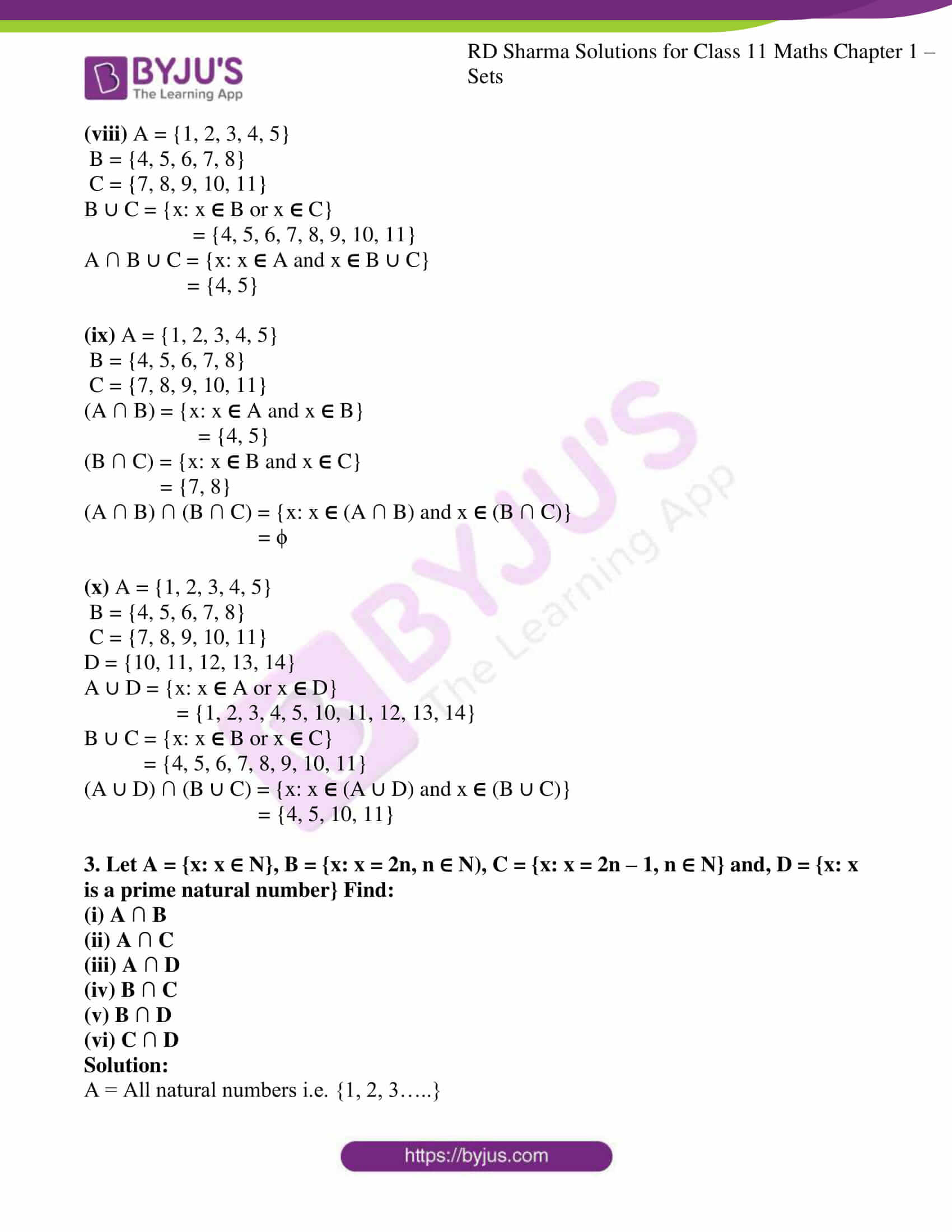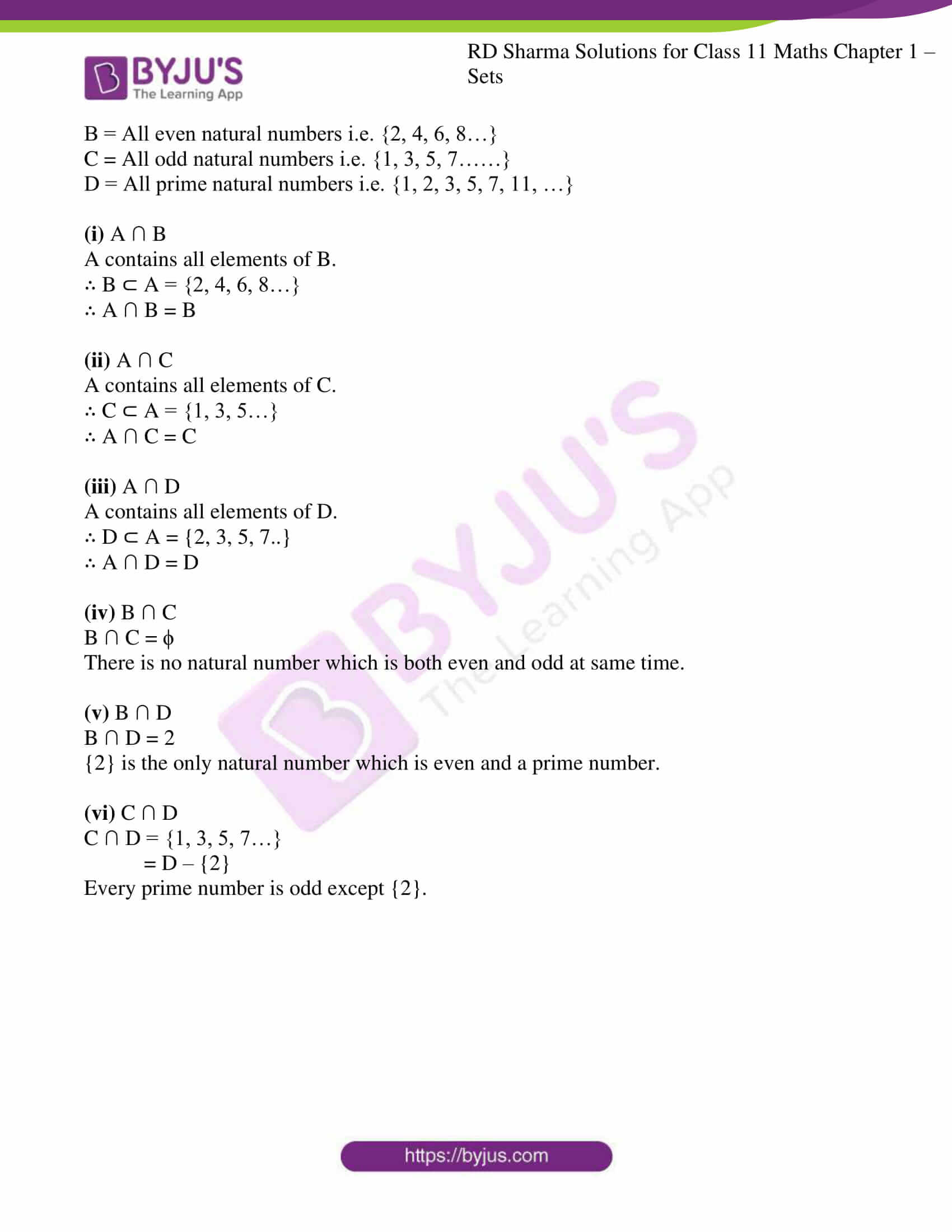# RD Sharma Solutions for Class 11 Chapter 1 - Sets Exercise 1.5

Sometimes a pictorial representation is very helpful in our thinking, so in Exercise 1.5 of Chapter 1, we deal with Venn diagrams and introduce some operations on sets to construct new sets from given ones. Union of sets, the intersection of sets, disjoint sets, the difference of sets, the symmetric difference of two sets, the complement of a set are also included in Venn diagrams. Students can self analyze the knowledge obtained about the chapter by solving the solutions which are formulated by our expert team. Students aspiring to score high marks are advised to download and practice the solutions on a daily basis. RD Sharma Class 11 Solutions are available in the pdf format which can be downloaded from the links given below.

## Download the Pdf of RD Sharma Solutions for Class 11 Maths Exercise 1.5 Chapter 1 – Sets### Access answers to RD Sharma Solutions for Class 11 Maths Exercise 1.5 Chapter 1 – Sets

1. If A and B are two sets such that A ⊂ B, then Find:
(i) A ⋂ B

(ii) A ⋃ B

Solution:

(i) A ∩ B

A ∩ B denotes A intersection B. Common elements of A and B consists in this set.

Given A ⊂ B, every element of A are already an element of B.

∴ A ∩ B = A

(ii) A ⋃ B

A ∪ B denotes A union B. Elements of either A or B or in both A and B consist in this set.

Given A ⊂ B, B is having all elements including elements of A.

∴ A ∪ B = B

2. If A = {1, 2, 3, 4, 5}, B = {4, 5, 6, 7, 8}, C = {7, 8, 9, 10, 11} and D = {10, 11, 12, 13, 14}. Find:
(i) A ∪ B
(ii) A ∪ C
(iii) B ∪ C
(iv) B ∪ D
(v) A ∪ B ∪ C
(vi) A ∪ B ∪ D
(vii) B ∪ C ∪ D
(viii) A ∩ (B ∪ C)
(ix) (A ∩ B) ∩ (B ∩ C)
(x) (A ∪ D) ∩ (B ∪ C).

Solution:

In general X ∪ Y = {a: a  X or a  Y}

X ∩ Y = {a: a  X and a  Y}.

(i) A = {1, 2, 3, 4, 5}

B = {4, 5, 6, 7, 8}

A ∪ B = {x: x  A or x  B}

= {1, 2, 3, 4, 5, 6, 7, 8}

(ii) A = {1, 2, 3, 4, 5}

C = {7, 8, 9, 10, 11}

A ∪ C = {x: x  A or x  C}

= {1, 2, 3, 4, 5, 7, 8, 9, 10, 11}

(iii) B = {4, 5, 6, 7, 8}

C = {7, 8, 9, 10, 11}

B ∪ C = {x: x  B or x  C}

= {4, 5, 6, 7, 8, 9, 10, 11}

(iv) B = {4, 5, 6, 7, 8}

D = {10, 11, 12, 13, 14}

B ∪ D = {x: x  B or x  D}

= {4, 5, 6, 7, 8, 10, 11, 12, 13, 14}

(v) A = {1, 2, 3, 4, 5}

B = {4, 5, 6, 7, 8}

C = {7, 8, 9, 10, 11}

A ∪ B = {x: x  A or x  B}

= {1, 2, 3, 4, 5, 6, 7, 8}

A ∪ B ∪ C = {x: x  A ∪ B or x  C}

= {1, 2, 3, 4, 5, 6, 7, 8, 9, 10, 11}

(vi) A = {1, 2, 3, 4, 5}

B = {4, 5, 6, 7, 8}

D = {10, 11, 12, 13, 14}

A ∪ B = {x: x  A or x  B}

= {1, 2, 3, 4, 5, 6, 7, 8}

A ∪ B ∪ D = {x: x  A ∪ B or x  D}

= {1, 2, 3, 4, 5, 6, 7, 8, 10, 11, 12, 13, 14}

(vii) B = {4, 5, 6, 7, 8}

C = {7, 8, 9, 10, 11}

D = {10, 11, 12, 13, 14}

B ∪ C = {x: x  B or x  C}

= {4, 5, 6, 7, 8, 9, 10, 11}

B ∪ C ∪ D = {x: x  B ∪ C or x  D}

= {4, 5, 6, 7, 8, 9, 10, 11, 12, 13, 14}

(viii) A = {1, 2, 3, 4, 5}

B = {4, 5, 6, 7, 8}

C = {7, 8, 9, 10, 11}

B ∪ C = {x: x  B or x  C}

= {4, 5, 6, 7, 8, 9, 10, 11}

A ∩ B ∪ C = {x: x  A and x  B ∪ C}

= {4, 5}

(ix) A = {1, 2, 3, 4, 5}

B = {4, 5, 6, 7, 8}

C = {7, 8, 9, 10, 11}

(A ∩ B) = {x: x  A and x  B}

= {4, 5}

(B ∩ C) = {x: x  B and x  C}

= {7, 8}

(A ∩ B) ∩ (B ∩ C) = {x: x  (A ∩ B) and x  (B ∩ C)}

= ϕ

(x) A = {1, 2, 3, 4, 5}

B = {4, 5, 6, 7, 8}

C = {7, 8, 9, 10, 11}

D = {10, 11, 12, 13, 14}

A ∪ D = {x: x  A or x  D}

= {1, 2, 3, 4, 5, 10, 11, 12, 13, 14}

B ∪ C = {x: x  B or x  C}

= {4, 5, 6, 7, 8, 9, 10, 11}

(A ∪ D) ∩ (B ∪ C) = {x: x  (A ∪ D) and x  (B ∪ C)}

= {4, 5, 10, 11}

3. Let A = {x: x ∈ N}, B = {x: x = 2n, n ∈ N), C = {x: x = 2n – 1, n ∈ N} and, D = {x: x is a prime natural number} Find:
(i) A ∩ B
(ii) A ∩ C
(iii) A ∩ D
(iv) B ∩ C
(v) B ∩ D
(vi) C ∩ D

Solution:

A = All natural numbers i.e. {1, 2, 3…..}

B = All even natural numbers i.e. {2, 4, 6, 8…}

C = All odd natural numbers i.e. {1, 3, 5, 7……}

D = All prime natural numbers i.e. {1, 2, 3, 5, 7, 11, …}

(i) A ∩ B

A contains all elements of B.

∴ B ⊂ A = {2, 4, 6, 8…}

∴ A ∩ B = B

(ii) A ∩ C

A contains all elements of C.

∴ C ⊂ A = {1, 3, 5…}

∴ A ∩ C = C

(iii) A ∩ D

A contains all elements of D.

∴ D ⊂ A = {2, 3, 5, 7..}

∴ A ∩ D = D

(iv) B ∩ C

B ∩ C = ϕ

There is no natural number which is both even and odd at same time.

(v) B ∩ D

B ∩ D = 2

{2} is the only natural number which is even and a prime number.

(vi) C ∩ D

C ∩ D = {1, 3, 5, 7…}

= D – {2}

Every prime number is odd except {2}.